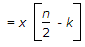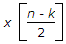Top

# Signals and Systems

1.

In Laplace transform, multiplication by e-at in time domain becomes

£e-at f(t) = F(s + a).

Enter details here

2.

ROC of sequence x[n] = (3)n ∪[n] + (4)n ∪[- n - 1]

No Explanation.

Enter details here

3.

The property is not valid for basic singularity function is

Singularity function is discontinuous at origin like ∪(t), δ(t).

Enter details here

4.

A voltage V(t) is a Gaussian ergodic random process with a mean of zero and a variance of 4 volt2. If it is measured by a dc meter. The reading will be

Enter details here

5.

In an ac circuit the fundamental component of current wave lags the corresponding voltage wave by 20°. The third harmonic component of current wave lags the corresponding voltage by an angle.

No explanation.

Enter details here

6.

Which one is a causal system?

For causal output must depend upon Present and past not on future.

Enter details here

7.

Which one is time invariant system?

For time invariant system

y(nk) = y(n - k), where y(nk) mean shift the input by n - k, while y(n - k) mean change all the n

(a) y[n] = x[2n]

⇒ y(nk) = x[2n - k]

y(n - k) = x[2(n - k)]

y(nk) ≠ y(n - k) Time variant

(b) y[n] = x[nx[n - 1]

y[nk] = x[n - kx[n - k - 1]

y[n - k] = x[n - kx[n - k - 1]

y[nk] = x[n - k] Time Invariant

(c) y[n] = x[n/2] ⇒ y[nk⇒ y[n - ky[nk] ≠ y[n - k] Time variant.

Enter details here

8.

δ(t) is a

Because Unit step is a Power signal. So By trignometric identifies d(t) also power.

Enter details here

9.

The ROC of sequence in the Z.T. of sequence x[n] = an ∪ [n] is

The simplest method to find the ROC. Put denominator to greater than zero.

Enter details here

10.

The signal defined by the equations f(t) = 0 for t < 0>f(t) = E for 0 ≤ t ≤ a and f(t) = 0 for t > a is